SVG 1.2 - 27 October 2004

# 10 Rendering model

SVG 1.2 updates the rendering model and rendering operations of SVG 1.1.

## 10.1 Enhanced Alpha Compositing

### 10.1.1 Introduction

SVG 1.2 supports the following clipping/masking features:

• alpha compositing, which may be used each time a new element is placed on the canvas. The operation specified determines the combination of the source color and alpha, and the destination color and alpha.
• clipping paths, which use any combination of path, text and basic shapes to serve as the outline of a (in the absence of anti-aliasing) 1-bit mask, where everything on the "inside" of the outline is allowed to show through but everything on the outside is masked out
• masks, which are container elements which can contain graphics elements or other container elements which define a set of graphics that is to be used as a semi-transparent mask for compositing foreground objects into the current background.

Note that masking with an element containing only color components with full luminance (e.g. r=g=b=1) will produce the equivalent result to compositing using the src-in or dst-in operators.

### 10.1.2 Alpha compositing

Graphics elements are composited onto the elements already rendered on the canvas based on an extended Porter-Duff compositing model, in which the resulting color and opacity at any given pixel on the canvas depend on the 'comp-op' specified. The base set of 12 Porter-Duff operations shown below always result in a value between zero and one, and as such, no clamping of output values is required.

In addition to the base set of 12 Porter-Duff operations, a number of blending operations are supported. These blending operations are extensions of the base Porter-Duff set and provide enhanced compositing behavior. The extended operations may result in color and opacity values outside the range zero to one. The opacity value should be clamped between zero and one inclusive, and the pre-multiplied color value should be clamped between zero and the opacity value inclusive.

The following diagram shows the four different regions of a single pixel that are considered when compositing.Depending on the compositing operation the resultant pixel includes input from one or more of the regions in the above diagram. For the regions where only source or destination are present, a choice of including or not including the input is available. For the region where both are present, various options are available for the combination of input data.

For groups containing compositing operators, the operation used to composite the group onto the canvas is the comp-op property of the container element itself. Other properties on container elements, such as opacity, specify operations that are performed after the children have been combined and before the group is composited onto the background. The enable-background and knock-out properties specify the state the group buffer is initialized to prior to use, any modification to the compositing of the group's children, and in some cases a post rendering step to be performed after rendering the children and prior to any other post rendering steps.

Implementation note: Various container elements calculate their bounds prior to rendering. For example, rendering a group generally requires an off-screen buffer, and the size of the buffer is determined by calculating the bounds of the objects contained within the group. SVG 1.0 implementations generally calculated the bounds of the group by calculating the union of the bounds of each of the objects within the group. Depending on the compositing operations used to combine objects within a group, the bounds of the group may be reduced, and so, reduce the memory requirements. For example, if a group contains two objects - object A 'in' object B - then the bounds of the group would be the intersection of the bounds of objects A and B as opposed to the union of their bounds.

While container elements are defined as requiring a buffer to be generated, it is often the case that a user agent using various optimizations can choose not to generate this buffer. For example, a group containing a single object could be directly rendered onto the background rather than into a buffer first.

The following variables are used to describe the components of the background, group and extra opacity channel buffers.

```Sc              Non-premultiplied source color component
Sca             Premultiplied source color component
Sra Sga Sba     Premultiplied source color component
Sa              Source opacity component
Dc              Non-premultiplied destination color component
Dca             Premultiplied destination color component
Dra Dga Dba     Non-premultiplied destination color component
Da              Destination opacity component
Da(d)           Extra opacity buffer containing the percentage
of the background channel in the group buffer.
D<n>            Destination buffer <n> where the background is 0,
groups in the top level svg element 1, nested groups
2 and so forth
D'              The results of the destination post a compositing step```

The operation used to place objects onto the background is as follows:

```      Dca' = f(Sc, Dc).Sa.Da  + Y.Sca.(1-Da)  + Z.Dca.(1-Sa)
Da'  =         X.Sa.Da  + Y.Sa.(1-Da)   + Z.Da.(1-Sa)```

Depending on the compositing operation, the above equation is resolved into an equation in terms of pre-multiplied values prior to rendering. The following are specified for each compositing operation:

`      X, Y, Z, f(Sc, Dc) `

defined as:

```f(Sc,Dc)    The intersection of the opacity of the source and destination
multiplied by some function of the color. (Used for color)

X           The intersection of the opacity of the source and destination.
(Used for opacity)

Y           The intersection of the source and the inverse of the destination.

Z           The intersection of the inverse of the source and the destination.```

Depending on the compositing operation, each of the above values may or may not be used in the generation of the destination pixel value.

### 10.1.3 The clip-to-self property

clip-to-self
 Value: true | false | inherit Initial: false Applies to: container elements and graphics elements Inherited: no Percentages: N/A Media: visual Animatable: yes

The clip-to-self property determines if the object effects pixels not covered by the object. Some compositing operations effect pixels where the source graphic is completely transparent.

For regions not covered by the source graphic, one of two operations can be performed.

1. Setting clip-to-self to true means that compositing an object only effects the pixels covered by the object.
2. Setting clip-to-self to false means that compositing an object effects all pixels on the canvas by compositing completely transparent source onto the destination for areas not covered by the object.

Note that most compositing operations do not remove the destination and as such for these operations, the clip-to-self property has no effect. The compositing operations that remove background are described in the comp-op property diagram. They are the operation which remove the right-hand blue region in each diagram. They are clear, src, src-in, dst-in, src-out and dst-atop. For all other operators the clip-to-self property has no effect.

The clip-to-self property provides compatibility with Java2D.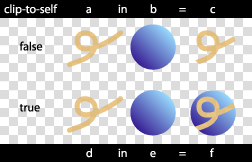View this image as SVG (SVG 1.2 enabled browsers only)

Container elements where the clip-to-self property is set to true only effect the pixels within the extent of the container element. For example, if a container element contains two circles, and the container element has the clip-to-self property set to true, then nothing outside the circles is effected. To perform this operation, the renderer needs to keep track of the extent of each of the elements within the container element and ensure that nothing other than the elements is modified. This can be produced by converting each object to a clipping path and unioning the clipping paths together to produce a clipping path that represents the extent of all the elements within the container element. Where a container element contains nested container elements, the operation is performed within the sub-container elements to produce the final path. When the group is composited onto the page, it is composited through the clipping path generated and thus nothing outside the extent of all the elements within the container element is modified.

For filled and stroked shapes and text, the object is directly converted to a clipping path. For images and filters, the bounds of the object are converted to a clipping region.

For some container elements where the clip-to-self property is set to false, the container element might effect the background outside bounds of the container element.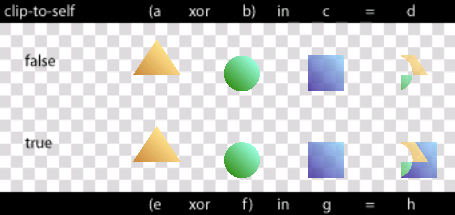View this image as SVG (SVG 1.2 enabled browsers only)

### 10.1.4 The enable-background property

enable-background
 Value: accumulate | new [ x y width height ] | inherit Initial: accumulate Applies to: container elements Inherited: no Percentages: N/A Media: visual Animatable: no

For a container element with enable-background set to "new", the container element's buffer is initially cleared to transparent. This buffer is treated as the canvas for the complex group's children. When the complete contents of the container element are rendered onto the buffer, the buffer is composited onto the canvas using the container element's specified compositing operation.

For a container element with enable-background set to "accumulate", the corresponding area of the canvas is copied into the container element's buffer. A second buffer which has only an opacity channel is also created. This buffer Da(d) stores the percentage of the background in the group buffer and is initially opaque. The group buffer is treated as the canvas for the children of the group as usual. Additionally, as objects are placed into the group buffer, they are also placed into the Da(d) buffer using one of the operations listed below. Before the group buffer is composited onto the canvas, any remaining background color in the group buffer is removed using the values in the Da(d) buffer. Other post rendering steps such as the opacity are performed after this step, and before compositing the result onto the canvas.

For groups with an enable-background value set to accumulate, the compositing operation used to place the group onto the background is modified. The operation will apply any reduction to the background caused by the objects.

When drawing elements within a container element with enable-background set to "accumulate", the standard equations as listed below are used to draw the object into the group buffer. Depending on the compositing operation, one of two operations listed below are used to draw the object into the extra transparency buffer Da(d).

For the operations clear, src, src-in, dst-in, src-out and dst-atop:

`Da(d)' = 0`

For all other compositing operations:

`Da(d)' = Da(d).(1 - Sa)`

Once the contents of a container element are rendered into the container element's buffer and before operations such as opacity or filters are applied to the buffer, the remaining background is removed from the container element's buffer using the following operations:

```Dca1'   = Dca1 - Dca0.Da1(d)
Da1'    = Da1 - Da0.Da1(d)```

At this point Da1(d) should be inverted. The inverted Da1(d) represents the amount of data to be removed from the background when placing the container element onto the background.

`Da1(d)' = 1 - Da1(d)`

The next operation to perform is the application of opacity or filters to the container element's buffer. During this step, the operation(s) performed on Da1 should also be performed on Da1(d).

When compositing the group buffer onto the background, rather than the standard compositing operation listed above, the following operations should be used:

```Dca0' = f(Dc1,Dc0).Da1.Da0 + Y.Dca1.(1-Da0) + Z.Dca0.(1-Da1(d))
Da0'  =          X.Da1.Da0 + Y.Da1.(1-Da0)  + Z.Da0.(1-Da1(d))```

Note that the last term in the above equations uses the Da(d) buffer rather than Da.

Elements containing a comp-op property value of clear, src, dst, dst-over, src-in, dst-in, src-out, dst-out, src-atop, dst-atop, xor can potentially reduce the opacity of the destination and are only valid where one of the element's ancestorial container element has the enable-background property set to new. For elements without an ancestor with the enable-background property set to "new" these operations are technically an error. A user agent should ignore the operation specified and render the element using the src-over compositing operation.

Filters have access to the nearest ancestor group's buffer through the BackgroundImage and BackgroundAlpha images. The buffer created for the ancestor group element of the element referencing the filter, is passed to the filter. Where no ancestors of the element referencing the filter contain an enable-background property value of new, transparent black is passed as input to the filter.

The optional x, y, width, height parameters on the new value indicate the subregion of the container element's user space where input filters have access to the background image. These parameters enable the SVG user agent potentially to allocate smaller temporary image buffers than the effective bounds of the container element. Thus, the values x, y, width, height act as a clipping rectangle on the background image canvas. Negative values for width or height are an error. If not all of the x, y, width and height values or if either width or height are specified as zero then the BackgroundImage and BackgroundAlpha are processed as if enable-background property was set to accumulate.

While container elements are defined as requiring a buffer to be generated, it is often the case that a user agent using various optimizations can choose not to generate this buffer. For example, a group containing a single object could be directly rendered onto the background rather than into a buffer first.

Where a filter references an area of the background image outside the area specified by x, y, width, height, transparent is passed to the filter.View this image as SVG (SVG 1.2 enabled browsers only)

### 10.1.5 The knock-out property

knock-out
 Value: true | false | inherit Initial: false Applies to: container elements Inherited: no Percentages: N/A Media: visual Animatable: no

For a complex group where the knock-out property is set, the buffer is created. The initial contents of the buffer and whether a secondary opacity channel is created depends on the value of the enable-background property. For each object within the container element, the object color and opacity replaces that of other objects within the container element, rather than overlaying it. In effect, the destination input to the compositing operations for the complex group's children is the original contents of the buffer, rather than the current buffer for the complex group.

For knock-out = false:

```      Dca1' = f(Sca, Sa, Dca1, Da1)
Da1' = f(Sa, Da1) ```

For knock-out = true, enable-background = new:

```      Dca1' = f(Sca, Sa, 0, 0)
Da1' = f(Sa, 0) ```

For 'knock-out' =true ,'enable-background' = accumulate:

```      Dca1' = f(Sca, Sa, Dca0, Da0)
Da1' = f(Sa, Da0) ```

Note that an element in a knockout group that does not have the clip-to-self property set, in effect clears all prior elements in the group.View this image as SVG (SVG 1.2 enabled browsers only)

### 10.1.6 The comp-op property

comp-op
 Value: clear | src | dst | src-over | dst-over | src-in | dst-in | src-out | dst-out | src-atop | dst-atop | xor | plus | multiply | screen | overlay | darken | lighten | color-dodge | color-burn | hard-light | soft-light | difference | exclusion | inherit Initial: src-over Applies to: container elements and graphics elements Inherited: no Percentages: N/A Media: visual Animatable: yes

The comp-op property determines the compositing operation used when placing elements onto the canvas. The canvas contains color components and an optional alpha component. When placing new elements onto the canvas, the resulting pixel values on the canvas are calculated using the equations listed in the sections below.

The diagram below shows the sub-pixel regions output by each of the compositing operations.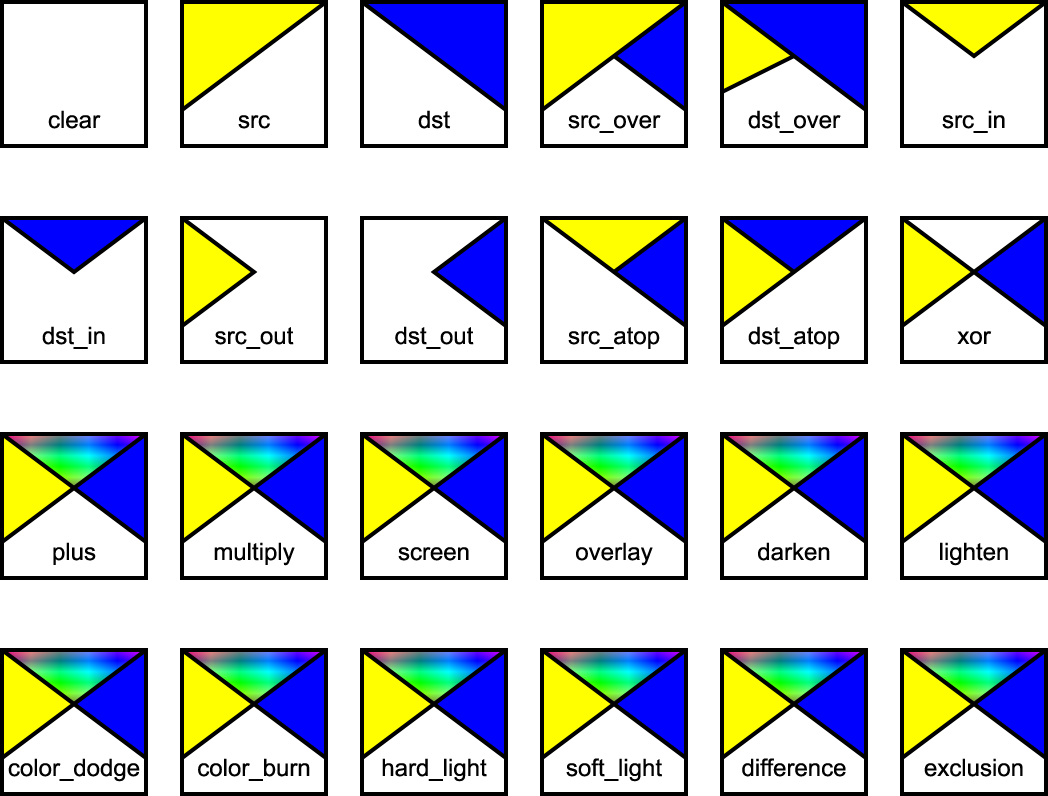For many of the operators listed below, the destination is modified in regions of the image where the source is completely transparent. Pixels that the source does not touch are considered transparent, and as such may be modified, depending on the compositing operation. As discussed in the previous section, the bounds of the parent container element can be optimized to save in memory usage and hence, pixel writing requirements. Once the bounds of the parent container element have been determined, each element can only affect the pixels within those bounds.

The following operators change pixels where the source is transparent: clear src src-in dst-in src-out dst-atop

The user agent may be required to create a backing store in which to generate a container element. The size of the backing store for a container element using the default compositing operator src-over is simply the union of the bounds of the sub-elements of the container element. When other compositing operators are used, the bounds of the container element are determined using the compositing operator diagram above. Starting with an empty bounds, the compositing operator specifies that the bounds of each successive object within the container element either replaces the result or is unioned with the result or is intersected with the result. For most compositing operators the bounds are unioned with the result. For the "clear" composite the current result is set to empty. For src, src-out and dst-atop, the bounds are set to the source bounds. For dst, dst-out and src-atop, the bounds are left unchanged. For src-in and dst-in the bounds are intersected with the result.

All color components listed below refer to color component information pre-multiplied by the corresponding alpha value. The following identifiers have the attached meaning in the equations below:

```      Sc  - The source element color value.
Sa  - The source element alpha value.
Dc  - The canvas color value prior to compositing.
Da  - The canvas alpha value prior to compositing.
Dc' - The canvas color value post compositing.
Da' - The canvas alpha value post compositing. ```
clear
Both the color and the alpha of the destination are cleared. Neither the source nor the destination are used as input.
```f(Sc,Dc) = 0
X        = 0
Y        = 0
Z        = 0

Dca' = 0
Da'  = 0```
src
The source is copied to the destination. The destination is not used as input.
```f(Sc,Dc) = Sc
X        = 1
Y        = 1
Z        = 0

Dca' = Sca.Da + Sca.(1 - Da)
= Sca
Da'  = Sa.Da + Sa.(1 - Da)
= Sa ```
dst
The destination is left untouched.
```f(Sc,Dc) = Dc
X        = 1
Y        = 0
Z        = 1

Dca' = Dca.Sa + Dca.(1 - Sa)
= Dca
Da'  = Da.Sa + Da.(1 - Sa)
= Da ```
src-over
The source is composited over the destination.
```f(Sc,Dc) = Sc
X        = 1
Y        = 1
Z        = 1

Dca' = Sca.Da + Sca.(1 - Da) + Dca.(1 - Sa)
= Sca + Dca.(1 - Sa)
Da'  = Sa.Da + Sa.(1 - Da) + Da.(1 - Sa)
= Sa + Da - Sa.Da ```

The following diagram shows src-over compositing:View this image as SVG (SVG 1.2 enabled browsers only)

dst-over
The destination is composited over the source and the result replaces the destination.
```f(Sc,Dc) = Dc
X        = 1
Y        = 1
Z        = 1

Dca' = Dca.Sa + Sca.(1 - Da) + Dca.(1 - Sa)
= Dca + Sca.(1 - Da)
Da'  = Da.Sa + Sa.(1 - Da) + Da.(1 - Sa)
= Sa + Da - Sa.Da ```
src-in
The part of the source lying inside of the destination replaces the destination.
```
f(Sc,Dc) = Sc
X        = 1
Y        = 0
Z        = 0

Dca' = Sca.Da
Da'  = Sa.Da ```

The following diagram shows src-in compositing:View this image as SVG (SVG 1.2 enabled browsers only)

dst-in
The part of the destination lying inside of the source replaces the destination.
```f(Sc,Dc) = Dc
X        = 1
Y        = 0
Z        = 0

Dca' = Dca.Sa
Da'  = Sa.Da ```
src-out
The part of the source lying outside of the destination replaces the destination.
```f(Sc,Dc) = 0
X        = 0
Y        = 1
Z        = 0

Dca' = Sca.(1 - Da)
Da'  = Sa.(1 - Da) ```

The following diagram shows src-out compositing: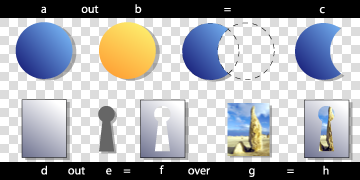View this image as SVG (SVG 1.2 enabled browsers only)

dst-out
The part of the destination lying outside of the source replaces the destination.
```f(Sc,Dc) = 0
X        = 0
Y        = 0
Z        = 1

Dca' = Dca.(1 - Sa)
Da'  = Da.(1 - Sa) ```
src-atop
The part of the source lying inside of the destination is composited onto the destination.
```f(Sc,Dc) = Sc
X        = 1
Y        = 0
Z        = 1

Dca' = Sca.Da + Dca.(1 - Sa)
Da'  = Sa.Da + Da.(1 - Sa)
= Da```

The following diagram shows src-atop compositing: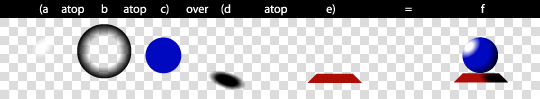View this image as SVG (SVG 1.2 enabled browsers only)

dst-atop

The part of the destination lying inside of the source is composited over the source and replaces the destination.

```f(Sc,Dc) = Dc
X        = 1
Y        = 1
Z        = 0

Dca' = Dca.Sa + Sca.(1 - Da)
Da'  = Da.Sa + Sa.(1 - Da)
= Sa ```
xor
The part of the source that lies outside of the destination is combined with the part of the destination that lies outside of the source.
```f(Sc,Dc) = 0
X        = 0
Y        = 1
Z        = 1

Dca' = Sca.(1 - Da) + Dca.(1 - Sa)
Da'  = Sa.(1 - Da) + Da.(1 - Sa)
= Sa + Da - 2.Sa.Da ```

The following compositing operators add blending of source and destination colors beyond the base 12 Porter-Duff operations. The behavior of these operators necessitates clamping of the output values after compositing.

plus

The source is added to the destination and replaces the destination. This operator is useful for animating a dissolve between two images.

```f(Sc,Dc) = Sc + Dc
X        = 1
Y        = 1
Z        = 1

Dca' = Sca.Da + Dca.Sa + Sca.(1 - Da) + Dca.(1 - Sa)
= Sca + Dca
Da'  = Sa.Da + Da.Sa + Sa.(1 - Da) + Da.(1 - Sa)
= Sa + Da ```
multiply

The source is multiplied by the destination and replaces the destination. The resultant color is always at least as dark as either of the two constituent colors. Multiplying any color with black produces black. Multiplying any color with white leaves the original color unchanged.

```f(Sc,Dc) = Sc.Dc
X        = 1
Y        = 1
Z        = 1

Dca' = Sca.Dca + Sca.(1 - Da) + Dca.(1 - Sa)
Da'  = Sa.Da + Sa.(1 - Da) + Da.(1 - Sa)
= Sa + Da - Sa.Da ```

The following diagram shows multiply compositing:View this image as SVG (SVG 1.2 enabled browsers only)

screen

The source and destination are complemented and then multiplied and then replace the destination. The resultant color is always at least as light as either of the two constituent colors. Screening any color with white produces white. Screening any color with black leaves the original color unchanged.

```f(Sc,Dc) = Sc + Dc - (Sc.Dc)

X        = 1
Y        = 1
Z        = 1

Dca' = (Sca.Da + Dca.Sa - Sca.Dca) + Sca.(1 - Da) + Dca.(1 - Sa)
= Sca + Dca - Sca.Dca
Da'  = Sa + Da - Sa.Da ```

The following diagram shows screen compositing: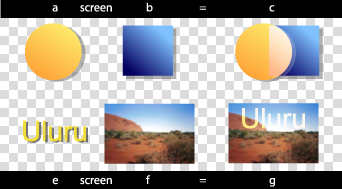View this image as SVG (SVG 1.2 enabled browsers only)

overlay

Multiplies or screens the colors, dependent on the destination color. Source colors overlay the destination whilst preserving its highlights and shadows. The destination color is not replaced, but is mixed with the source color to reflect the lightness or darkness of the destination.

```if 2.Dc < Da
f(Sc,Dc) = 2.Sc.Dc
otherwise
f(Sc,Dc) = 1 - 2.(1 - Dc).(1 - Sc)
X        = 1
Y        = 1
Z        = 1

if 2.Dca < Da
Dca' = 2.Sca.Dca + Sca.(1 - Da) + Dca.(1 - Sa)
otherwise
Dca' = Sa.Da - 2.(Da - Dca).(Sa - Sca) + Sca.(1 - Da) + Dca.(1 - Sa)

Da' = Sa + Da - Sa.Da```

The following diagram shows overlay compositing:View this image as SVG (SVG 1.2 enabled browsers only)

darken

Selects the darker of the destination and source colors. The destination is replaced with the source when the source is darker, otherwise it is left unchanged.

```f(Sc,Dc) = min(Sc,Dc)
X        = 1
Y        = 1
Z        = 1

Dca' = min(Sca.Da, Dca.Sa) + Sca.(1 - Da) + Dca.(1 - Sa)
Da'  = Sa + Da - Sa.Da

or

if Sca.Da < Dca.Sa
src-over()
otherwise
dst-over()```

The following diagram shows darken compositing:View this image as SVG (SVG 1.2 enabled browsers only)

lighten

Selects the lighter of the destination and source colors. The destination is replaced with the source when the source is lighter, otherwise it is left unchanged.

```f(Sc,Dc) = max(Sc,Dc)
X        = 1
Y        = 1
Z        = 1

Dca' = max(Sca.Da, Dca.Sa) + Sca.(1 - Da) + Dca.(1 - Sa)
Da'  = Sa + Da - Sa.Da

or

if Sca.Da > Dca.Sa
src-over()
otherwise
dst-over() ```

The following diagram shows lighten compositing: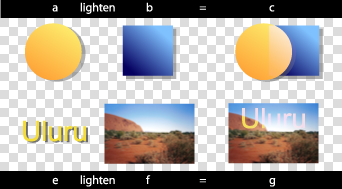View this image as SVG (SVG 1.2 enabled browsers only)

color-dodge

Brightens the destination color to reflect the source color. Painting with black produces no change.

```if Sc + Dc >= 1
f(Sc,Dc) = 1
otherwise
f(Sc,Dc) = Dc.1/(1-Sc)
X        = 1
Y        = 1
Z        = 1

if Sca.Da + Dca.Sa >= Sa.Da
Dca' = Sa.Da + Sca.(1 - Da) + Dca.(1 - Sa)
otherwise
Dca' = Dca.Sa/(1-Sca/Sa) + Sca.(1 - Da) + Dca.(1 - Sa)

Da'  = Sa + Da - Sa.Da ```

The following diagram shows color-dodge compositing: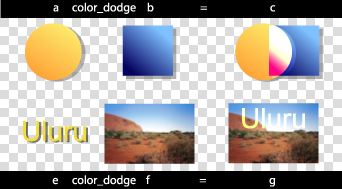View this image as SVG (SVG 1.2 enabled browsers only)

color-burn

Darkens the destination color to reflect the source color. Painting with white produces no change.

```if Sc + Dc <= 1
f(Sc,Dc) = 0
otherwise
f(Sc,Dc) = (Sc + Dc - 1)/Sc
X        = 1
Y        = 1
Z        = 1

if Sca.Da + Dca.Sa <= Sa.Da
Dca' = Sca.(1 - Da) + Dca.(1 - Sa)
otherwise
Dca' = Sa.(Sca.Da + Dca.Sa - Sa.Da)/Sca + Sca.(1 - Da) + Dca.(1 - Sa)

Da'  = Sa + Da - Sa.Da ```

The following diagram shows color-burn compositing:View this image as SVG (SVG 1.2 enabled browsers only)

hard-light

Multiplies or screens the colors, dependent on the source color value. If the source color is lighter than 0.5, the destination is lightened as if it were screened. If the source color is darker than 0.5, the destination is darkened, as if it were multiplied. The degree of lightening or darkening is proportional to the difference between the source color and 0.5. If it is equal to 0.5 the destination is unchanged. Painting with pure black or white produces black or white.

```if 2.Sc < 1
f(Sc,Dc) = 2.Sc.Dc
otherwise
f(Sc,Dc) = 1 - 2.(1 - Dc).(1 - Sc)
X        = 1
Y        = 1
Z        = 1

if 2.Sca < Sa
Dca' = 2.Sca.Dca + Sca.(1 - Da) + Dca.(1 - Sa)
otherwise
Dca' = Sa.Da - 2.(Da - Dca).(Sa - Sca) + Sca.(1 - Da) + Dca.(1 - Sa)

Da'  = Sa + Da - Sa.Da```

The following diagram shows hard-light compositing:View this image as SVG (SVG 1.2 enabled browsers only)

soft-light

Darkens or lightens the colors, dependent on the source color value. If the source color is lighter than 0.5, the destination is lightened. If the source color is darker than 0.5, the destination is darkened, as if it were burned in. The degree of darkening or lightening is proportional to the difference between the source color and 0.5. If it is equal to 0.5, the destination is unchanged. Painting with pure black or white produces a distinctly darker or lighter area, but does not result in pure black or white.

```if 2.Sc < 1
f(Sc,Dc) = Dc.(1 - (1 - Dc).(2.Sc - 1))
otherwise if 8.Dc <= 1
f(Sc,Dc) = Dc.(1 - (1 - Dc).(2.Sc - 1).(3 - 8.Dc))
otherwise
f(Sc,Dc) = (Dc + (Dc^(0.5) - Dc).(2.Sc - 1))
X        = 1
Y        = 1
Z        = 1

if 2.Sca < Sa
Dca' = Dca.(Sa - (1 - Dca/Da).(2.Sca - Sa)) + Sca.(1 - Da) + Dca.(1 - Sa)
otherwise if 8.Dca <= Da
Dca' = Dca.(Sa - (1 - Dca/Da).(2.Sca - Sa).(3 - 8.Dca/Da)) + Sca.(1 - Da) + Dca.(1 - Sa)
otherwise
Dca' = (Dca.Sa + ((Dca/Da)^(0.5).Da - Dca).(2.Sca - Sa)) + Sca.(1 - Da) + Dca.(1 - Sa)

Da'  = Sa + Da - Sa.Da ```

The following diagram shows soft-light compositing:View this image as SVG (SVG 1.2 enabled browsers only)

difference

Subtracts the darker of the two constituent colors from the lighter. Painting with white inverts the destination color. Painting with black produces no change.

```f(Sc,Dc) = abs(Dc - Sc)
X        = 1
Y        = 1
Z        = 1

Dca' = abs(Dca.Sa - Sca.Da) + Sca.(1 - Da) + Dca.(1 - Sa)
= Sca + Dca - 2.min(Sca.Da, Dca.Sa)
Da'  = Sa + Da - Sa.Da ```

The following diagram shows difference compositing:View this image as SVG (SVG 1.2 enabled browsers only)

exclusion

Produces an effect similar to that of 'difference', but appears as lower contrast. Painting with white inverts the destination color. Painting with black produces no change.

```
f(Sc,Dc) = (Sc + Dc - 2.Sc.Dc)
X        = 1
Y        = 1
Z        = 1

Dca' = (Sca.Da + Dca.Sa - 2.Sca.Dca) + Sca.(1 - Da) + Dca.(1 - Sa)
Da'  = Sa + Da - Sa.Da ```

These equations are approximations which are under review. Final equations may differ from those presented here.

The following diagram shows exclusion compositing:View this image as SVG (SVG 1.2 enabled browsers only)

## 10.2 Enhanced Transformations

SVG 1.2 extends the coordinate system transformations allowed on groups and elements to provide a method by which graphical objects can remain fixed in the viewport without being scaled.

The following summarizes the different transforms that are applied to a graphical object as it is rendered.

### 10.2.1 The user space transformation

The User Transform is the transformation that applies the user agent positioning controls to the coordinate system. This transform can be considered to be applied to a group that surrounds the outermost svg element of the document.

The user agent positioning controls consist of a translation (commonly referred to as the "pan"), a scale (commonly referred to as the "zoom") and a rotate.

```US = User Scale (currentScale on SVGSVGElement)
UP = User Pan (currentTranslate on SVGSVGElement)
UR = User Rotate (currentRotate on SVGSVGElement)```

The User Transform is the product of these component transformations.

```U = User Transform
= UP.US.UR```

### 10.2.2 ViewBox to Viewport transformation

SVG elements, such as the root svg, create their own viewport. The viewBox to viewport transformation is the transformation on an svg element that adjusts the coordinate system to take the viewBox and preserveAspectRatio attributes into account.

We use the following notation for a viewBox to viewport transformation:

`VB(svgId)`

The 'svgId' parameter is the value of the id attribute on a given svg element.

### 10.2.3 Element Transform Stack

All elements in an SVG document have a transform stack. This is the list of transforms that manipulate the coordinate system between the element and its nearest ancestor svg element.

We use the following notation for the Element Transform stack on a given element:

`TS(id)`

The 'id' parameter is the value of the id attribute on a given element. Note that for an svg element, TS() is the concatenation of transforms found on the ancestors of the svg element ( svg elements themselves do not have a transform attribute). Consequently, for an svg element, TS(svgId) is the stack of transforms that apply above the VB() transformation (if the svg element is not the root element).

Below is an example of the element transform stack:

```<svg id="root">
<g id="g" transform="scale(2)">
<rect id="r" transform="scale(4)"/>
<svg id="svg0" width="200" height="200" viewBox="0 0 100 100">
<rect id="r2" transform="scale(0.5)" />
</svg>
</g>
</svg>```

In this example, the transforms are:

```TS(g) = scale(2)
TS(r) = TS(g) . scale(4) = scale(8)
TS(r2) = scale(0.5)
TS(svg0) = scale(8)
VB(svg0) = scale(2)```

### 10.2.4 The Current Transform Matrix

Each element in the rendering tree has the concept of a Current Transform Matrix, or CTM. This is the product of all coordinate system transformations that apply to an element, effectively mapping the element into a coordinate system that is then transformed into device units by the user agent.

Consider the following example, with a rectangle having a set of ancestor svg elements with ids "svg-0" to "svg-n" ("svg-n" being the root).

```<svg id="svg-n">
...
<svg id="svg-n-1">
...
...
<svg id="svg-2">
...
<svg id="svg-1">
...
<svg id="svg-0">
...
<rect id="elt" .../>
</svg>
</svg>
</svg>
</svg>
</svg>```

With the above definitions, the CTM for the rectangle with id "elt" is:

```CTM(elt) = prod{i=0, i=n}(U[i].VB(svg[i]).TS(svg[i-1])).TS(elt)

Where prod{i=1, i=n}(f(i)) as:

prod{i=0, i=n}(f(i)) = f(n).f(n-1).f(n-2).[...].f(1).f(0)```

In the above definition, svg[n] refers to the element with the id "svg-n".

The TS() of a non-existent element is the identity transform. And:

`U[i] = Identity for i < n and U[n] = U.`

For example, with n=2, we have:

```<svg id="svg-2">
...
<svg id="svg-1">
...
<svg id="svg-0">
...
<rect id="elt" .../>
</svg>
</svg>
</svg>```

This produces the following transformations:

``` CTM(elt) = U.VB(svg).TS(svg))
.U.VB(svg).TS(svg)
.U.VB(svg).TS(elt)

= U.VB(svg).TS(svg).VB(svg).TS(svg).VB(svg).TS(elt)```

Note the important relationship between an element's CTM and its parent CTM, for elements which do not define a viewport:

` CTM(elt) = CTM(elt.parentElement).Txf(elt)`

where Txf(elt) is the transform defined by the element's transform attribute.

### 10.2.5 The ref() transform value

In SVG 1.2 the transform attribute has been extended to provide simple constrained transformations using the "ref()" attribute value. A transform attribute can have a value defined in SVG 1.1 or the new "ref" value. The two value types cannot be mixed.

The 'ref(svg, x, y)' transform evaluates to the inverse of the element's parent's CTM multiplied by the closest svg element's CTM (from top-most SVG viewport space to the closest svg element's user space) but exclusive of the closest svg element's user transform, if any. Note that only the outermost svg element can have a zoom/pan/rotate user transform. If the closest svg element is not the top-most svg element there is no user transform to exclude.

The x and y parameters are optional. If they are specified an additional translation is appended to the transform so that (0, 0) in the element's user space maps to (x, y) in the svg element's user space. If no x and y parameters are specified, no additional translation is applied.

Using the definitions provided above:

```Inverse of the parent's CTM: inv(CTM(elt.parentElement))

The closest svg element's user transform, exclusive of zoom,
pan and rotate transforms:
CTM(svg.parentElement).VB(svg)

Where CTM(svg.parentElement) evaluates to Identity if there
is no svg.parentElement element.```

In addition, the T(x, y) translation is such that:

`CTM(elt).(0, 0) = CTM(svg).(x, y)`

So the transform evaluates to:

`Txf(elt) = inv(CTM(elt.parentElement)).CTM(svg.parentElement).VB(svg).T(x, y)`

The element's CTM is:

```CTM(elt) = CTM(elt.parentElement).Txf(elt)
= CTM(svg.parentElement).VB(svg).T(x,y)```

In the following example, a small rectangle initially marks the middle of a line. The user agent viewport is a square with sides of 200 units.

```<svg id="root" viewBox="0 0 100 100">
<line x1="0" x2="100" y1="0" y2="100" />
<rect id="r" transform="ref(svg)"
x="45" y="45" width="10" height="10"/>
</svg> ```

In this case:

```Txf(r) = inv(CTM(r.parent)).CTM(root.parentElement).VB(root).T(x, y)

CTM(root.parentElement) evaluates to Identity.

T(x, y) evaluates to Identity because (x, y) is not specified

CTM(r) = CTM(r.parent).Txf(r)
= CTM(r.parent).inv(CTM(r.parent)).VB(root)
= VB(root)
= scale(2)```

Consequently, regardless of the user transform (currentTranslate, currentScale, currentRotate) the rectangle's coordinates in viewport space will always be: (45, 45, 10, 10)*scale(2) = (90, 90, 20, 20). Initially, the line is from (0, 0) to (200, 200) in the viewport coordinate system. If we apply a user agent zoom of 3 (currentScale = 3), the rectangle is still (90, 90, 20, 20) but the line is (0, 0, 600, 600) and the marker no longer marks the middle of the line.

In the following example a small rectangle always marks the middle of a line. Again, the user agent viewport is a square with sides of 200 units.

```<svg id="root" baseProfile="tiny" viewBox="0 0 100 100">
<line x1="0" x2="100" y1="0" y2="100"/>
<g id="g" transform="ref(svg, 50, 50)">
<rect id="r" x="-5" y="-5" width="10" height="10"/>
</g>
</svg>```

In this case:

```Txf(g) = inv(CTM(g.parent)).CTM(root.parentElement).VB(root).T(x,y)

CTM(root.parentElement) evaluates to Identity.

CTM(g) = CTM(g.parent).Txf(r)
= CTM(g.parent).inv(CTM(g.parent)).VB(root).T(x,y)
= VB(root).T(x,y)
= scale(2).T(x,y)
```

Initially, (50, 50) in the svg user space is (100, 100) in viewport space. Therefore:

```CTM(g).[0, 0] = CTM(root).[50, 50]
= scale(2).[50, 50]
= [100, 100]
and

scale(2).T(x,y) = [100, 100]

T(x,y) = translate(50, 50)```

If the user agent pan was (50, 80) (modifying currentTranslate) then we now have (50, 50) in the svg element's user space located at (150, 180) in the viewport space. This produces:

```CTM(g).[0, 0] = CTM(root).[50, 50]
= translate(50, 80).scale(2).[50, 50]
= [150, 180]

and

scale(2).T(x,y) = [150, 180]

T(x, y) = translate(75, 90)```

Therefore, regardless of the user transform, the rectangle marker will always overlap the middle of the line. Note that the marker will not rotate with the line (e.g., if currentRotate is set) and it will not scale either.

The example below contains nested svg elements:

```<svg id="fullSVGRoot">
<g id="hostGroup" transform="scale(2)">
<svg id="tinySVGRoot" baseProfile="tiny" width="400" height="400"
viewBox="0 0 100 100">
<rect id="r" transform="ref(svg)" x="5" width="90" y="5"
height="90" fill="red" />
</svg>
</g>
</svg> ```

If the element with id 'fullSVGRoot' has its zoom and pan transform modified, there is an effect on the rectangle's coordinate system. If the "hostGroup" transform is changed, there is an effect on the rectangle.

Using the above definitions, the transform attribute on 'r' evaluates to:

```Txf(r) = inv(CTM(elt.parentElement)).CTM(svg.parentElement).VB(svg).T(x,y)
= inv(CTM(tinySVGRoot)).CTM(tinySVGRoot.parentElement).VB(tinySVGRoot).T(x,y)
T(x,y) is identity.```

So, r's CTM is:

```CTM(r) = CTM(r.parent).Txf(r)
= CTM(tinySVGRoot).inv(CTM(tinySVGRoot)).
CTM(tinySVGRoot.parentElement).VB(tinySVGRoot)
= CTM(hostGroup).VB(tinySVGRoot)

CTM(hostGroup) = U.VB(fullSVGRoot).TS(hostGroup)

VB(fullSVGRoot) = Identity (no viewBox)
TS(hostGroup) = scale(2)
VB(tinySVGRoot) = scale(4)

CTM(r) = scale(2).scale(4)
= scale(8)```

Consequently, the rectangle is always displayed at (20, 20, 360, 360) in the tinySVGRoot viewport space. Since this viewport is subject to the 'fullSVGRoot' user transform, the rectangle will be affected by changes to the root svg's user transform.